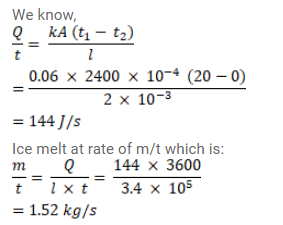# An ice box almost completely filled with ice at 0°C`
Question:

An ice box almost completely filled with ice at $0^{\circ} \mathrm{C}$ is dipped into a large volume of water at $20^{\circ} \mathrm{C}$. The box has walls of surface area $2400 \mathrm{~cm}^{2}$ thickness $2.0 \mathrm{~mm}$ and thermal conductivity $0.06 \mathrm{~W} / \mathrm{m}-{ }^{\circ} \mathrm{C}$ Calculate the rate at which the ice melts in the box. Latent heat of fusion of ice $=3.4 \times 10^{5} \mathrm{~J} / \mathrm{kg}$

Solution: